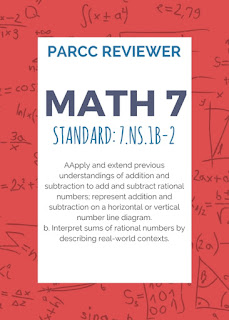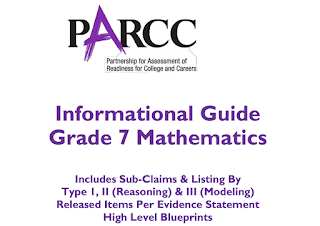## GED Practice

### Math 7 PARCC Reviewer 9 (7.NS.1b-2)

Sub-claim:
The students solve problems involving the Major Content with connections to the Standards for Mathematical Practice
Type of items: Type 1
Color Code: Peach
Evidence Statement Key: 7.NS.1b-2
Calculator: No
Point: 1 each (1:29)
Evidence Statement Text:
Apply and extend previous understandings of addition and subtraction to add and subtract rational numbers; represent addition and subtraction on a horizontal or vertical number line diagram. b. Interpret sums of rational numbers by describing real-world contexts.
Clarifications, limits, emphases, and other information intended to ensure appropriate variety in tasks:
1. Tasks require students to produce or recognize real-world contexts that correspond to given sums of rational numbers.
2. Tasks are not limited to integers.
3. Tasks do not require students to show in general that a number and its opposite have a sum of 0; for this aspect of 7.NS.1b-1, see 7.C.1.a and 7.C.2
Sample Questions (taken from PARCC’s Practice Tests and Released Items):
EOY Released Test Item 11. EOY Released Test Item #1
A meteorologist was monitoring the temperature outside in degrees Fahrenheit (°F) and wrote the expression 78 + ( - 6 ) - 5. Which statement best describes this expression?
a. The temperature started at 78°F and increased by 6°F.
Then the temperature decreased by 5°F.
b. The temperature started at 78°F and increased by 6°F.
Then the temperature increased by 5°F.
c. The temperature started at 78°F and decreased by 6°F.
Then the temperature decreased by 5°F.
b. The temperature started at 78°F and decreased by 6°F.
Then the temperature increased by 5°F.